Next: 1. Notation, Undefined Concepts Up: Introduction Previous: The Goal of the   Index

# Some General Remarks

The exercises in these notes are important. The proofs of many theorems will appear as exercises. You should work on as many exercises as time permits. Do not be discouraged if you cannot do some of the exercises the first time you try them. The important thing is that you should be able to do them after they have been discussed in class. The entertainments are supposed to be entertaining. If they do not entertain you, you can ignore them (unless your instructor is so entertained that one gets assigned as a homework problem). There are hints for selected problems at the end of the notes. Do not use them until you have spent some time on the problems. Any method you discover on your own is better than any method suggested in a hint.

The prerequisite for this course is a course in one-variable calculus. From the remarks made above you know that you cannot assume any facts from your calculus course, but many theorems are motivated by calculus. You should know that the derivative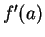of a function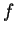at a pointis given by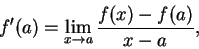and that this represents the slope of the tangent to the graph ofat the point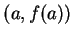. If you are not familiar with the rules for calculating the derivatives of the sine and cosine and exponential functions from an earlier course, our definitions of the sine and cosine and exponential will seem rather meaningless.

In these notes, informal set theory and logic are used. Axioms for set theory and logic can be given, and most mathematicians believe that all of the sorts of informal proofs that we give in the notes can, in principle, be justified by the axioms of set theory and logic. However the sort of informal set theory and logic we use here are typical of the methods used by workers in mathematical analysis at the start of the twenty-first century.

***

These notes are largely based on Joe Buhler's math 112 notes, used at Reed College in spring 1998. The material in Buhler's appendices has been expanded and put into the main text. There are more examples, and some proofs are given in more detail. I've added some pictures, because I think geometrically.

Entertainment 0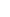Suppose three lines are given, having lengths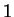,, and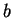.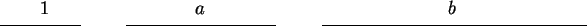a) Describe a compass-and-straightedge construction for a line segment of length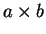.

b) Describe a compass-and-straightedge construction for a line segment of length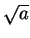.Next: 1. Notation, Undefined Concepts Up: Introduction Previous: The Goal of the   Index